##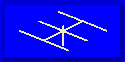ANTENNAS FROM THE GROUND UP### L. B. Cebik, W4RNLThe fundamental concepts and realities of Off-Center-fed (OCF) wire antennas developed in terms of 40-meter models apply to any other fundamental band with adjustments of height in terms of fractions of a wavelength. However, several folks have asked for more specifics for an 80-meter-based OCF in order to have reasonable expectations of performance.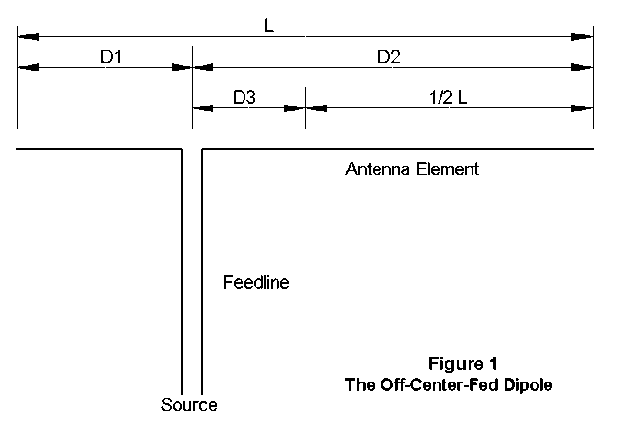For an 80-meter fundamental OCF , L will equal about 135' if the antenna is resonant. If the antenna is fed with parallel transmission line to an ATU, the exact length is not critical. However, for the sample patterns, it has been made resonant.

We shall sample an 80-meter OCF at 35' height, a typical amateur installation. The frequency of basic resonance is 3.5 MHz to ensure that harmonics fall within the amateur bands. However, pattern samples will cover all 8 amateur bands between 80 and 10 meters.

We shall also sample two points of antenna element-to-feeder junction: the 100-ohm and the 300-ohm points. The antenna dimensions for these two antennas are as follows:

• 100-ohm Connection: L = 135.25' D1 = 34.7' D2 = 100.55'

• 300-ohm connection: L = 135.67' D1 = 18.2' D2 = 117.47'

Interestingly, this same antenna raised to a height of 70' shows the following resonant characteristics:

• 100-ohm Connection: L = 135.7' D1 = 50.5' D2 = 85.2'

• 300-ohm Connection: L = 136.55' D1 = 24.1' D2 = 112.45'

35' and 70' are 1/8 and 1/4 wavelength heights, respectively, a region where we can expect large changes of antenna characteristics with small changes in height. The patterns and numbers associated with the antennas should thus be used with due caution. They are indicators, not absolutes.

For each pattern, a feedpoint impedance figure, Zi, is given. Zi is the impedance assuming that the radiation is confined to the antenna element. Since this assumption will be violated in most installations using parallel feedline directly connected to the antenna, the impedance figures are best treated as indicators of moderate or high impedances, not as numbers to be expected in reality. The actual impedance presented to the ATU will be a complex combination of the degree to which the feedline contributes to radiation and the transformation of the antenna impedance along the line.

#### 80 Meters: 3.6 MHz

100-ohm Connection: Zi = 120 + j100 ohms -- Elevation angle = 45 degrees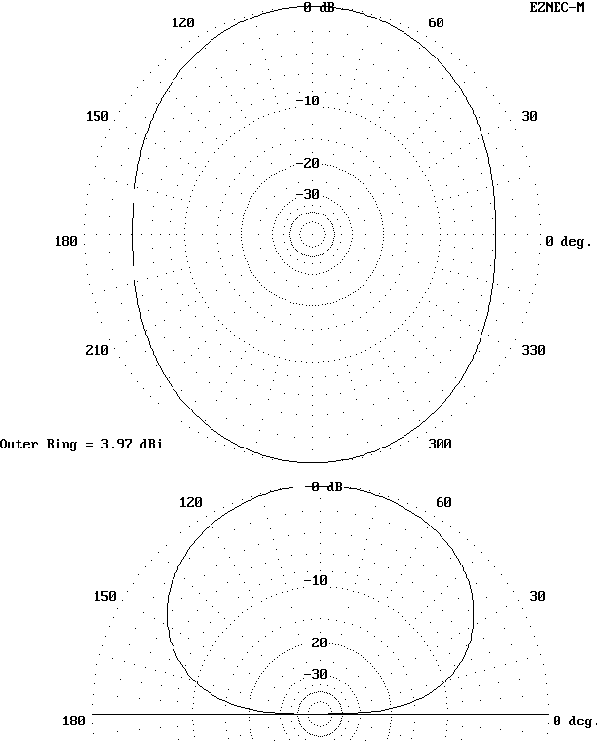Note: Since the actual elevation angle of maximum radiation is greater than 45 degrees for 80 and 40 meters, the azimuth patterns are taken at an elevation angle of 45 degrees.

300-ohm Connection: Zi = 455 + j310 ohms -- Elevation angle = 45 degrees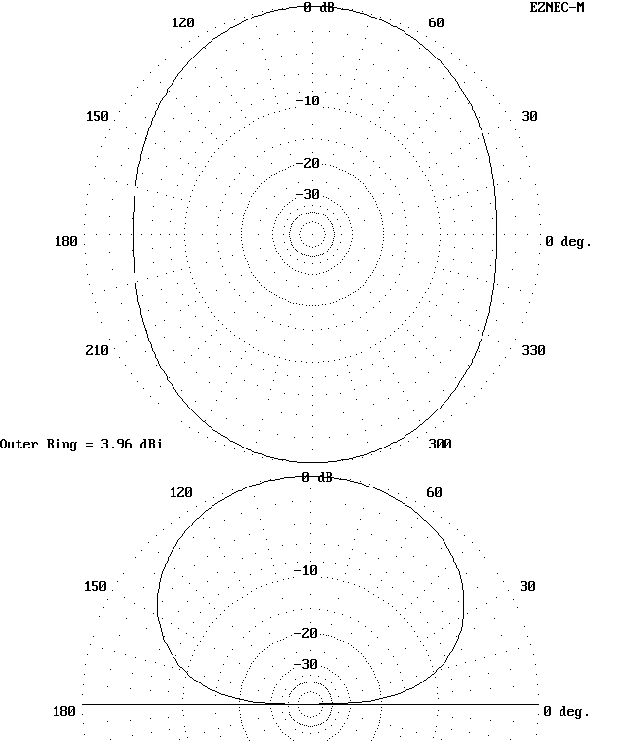#### 40 Meters: 7.15 MHz

100-ohm Connection: Zi = 105 + j15 ohms -- Elevation angle = 45 degrees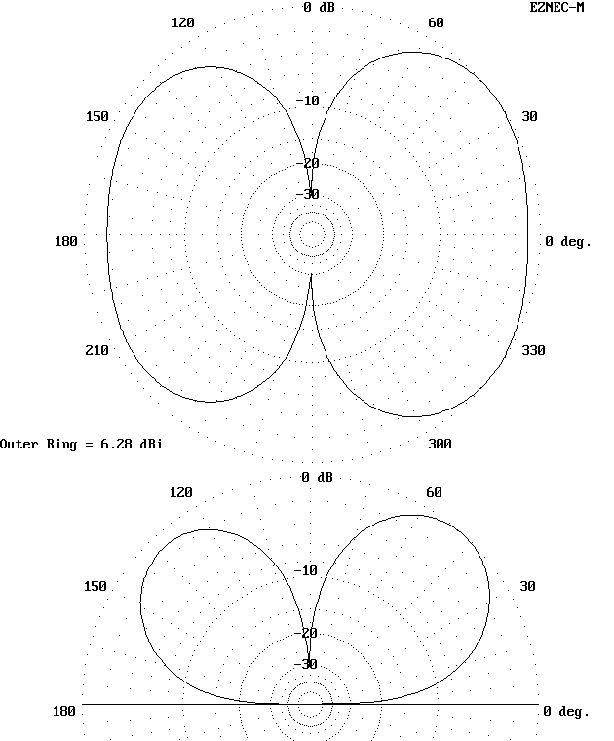Note: For a different slant on OCF antennas, see Chapter 3 of Bill Orr, W6SAI, HF Antenna Handbook. He presents some interesting variations on the versions studied here.

300-ohm Connection: Zi = 185 + j20 ohms -- Elevation angle = 45 degrees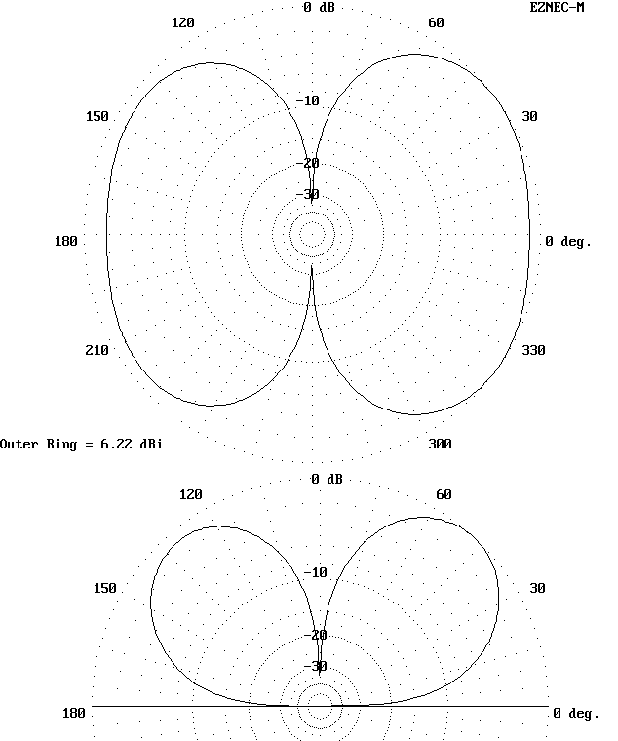#### 30 Meters: 10.1 MHz

100-ohm Connection: Zi = 690 - j890 ohms -- Elevation angle = 37 degrees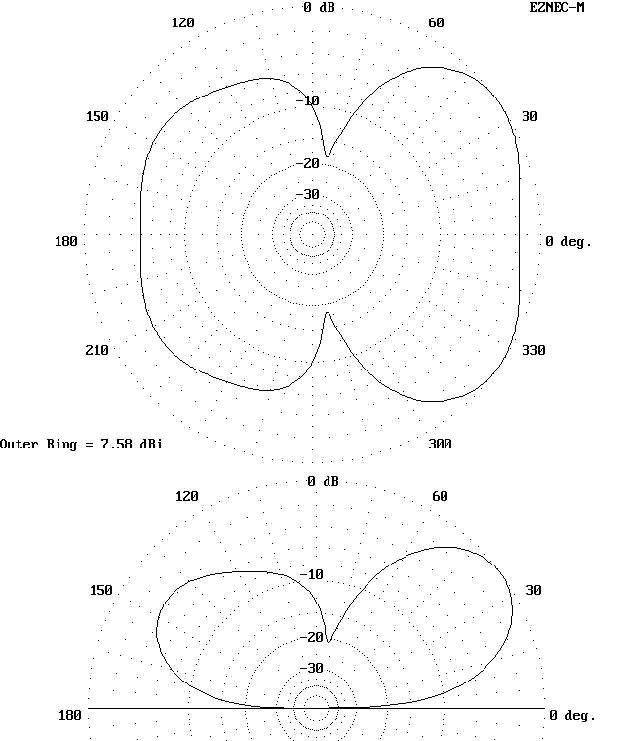Note that differences between the 100-ohm and the 300-ohm patterns are beginnig to appear with respect to both azimuth and elevation.

300-ohm Connection: Zi = 90 - j295 ohms -- Elevation angle = 36 degrees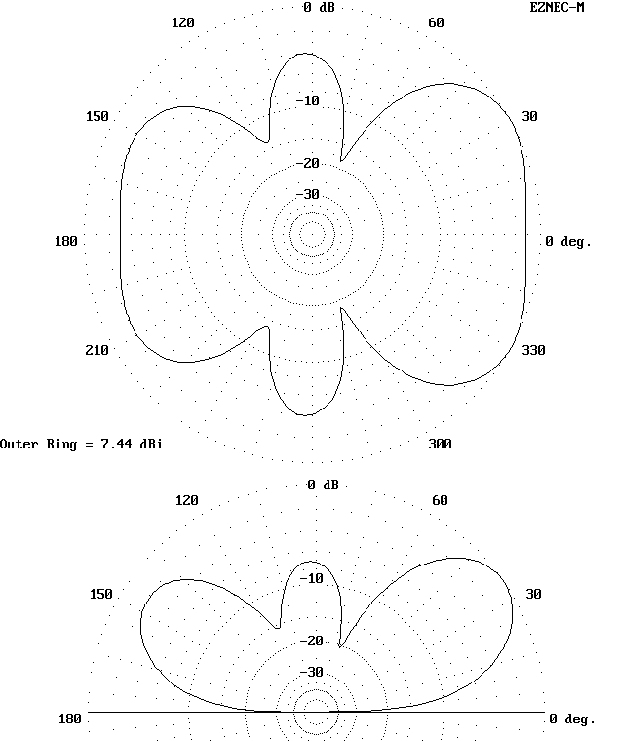#### 20 Meters: 14.15 MHz

100-ohm Connection: Zi = 3145 - j1355 ohms -- Elevation angle = 28 degrees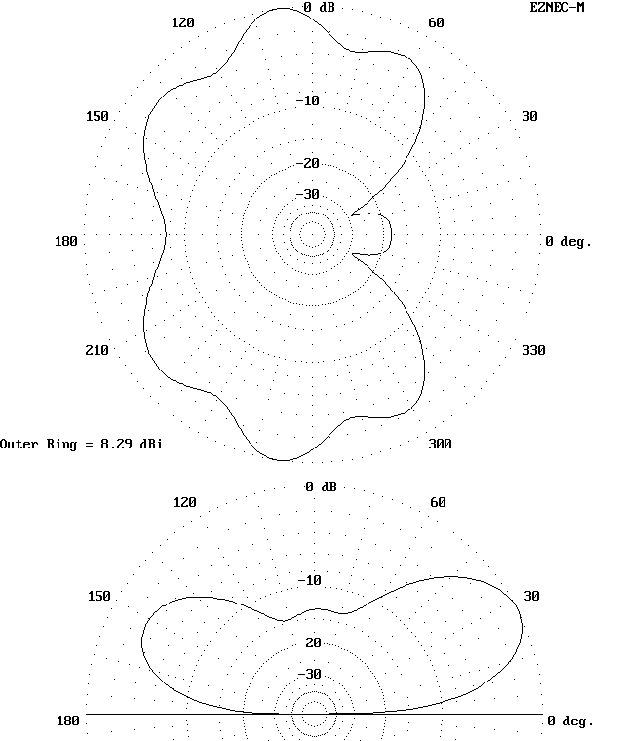At 20 meters, there is a wide divergence between 100-ohm and 300-ohm patterns, with the 100-ohm pattern more closely resembling the pattern of a center-fed antenna.

300-ohm Connection: Zi = 120 - j105 ohms -- Elevation angle = 25 degrees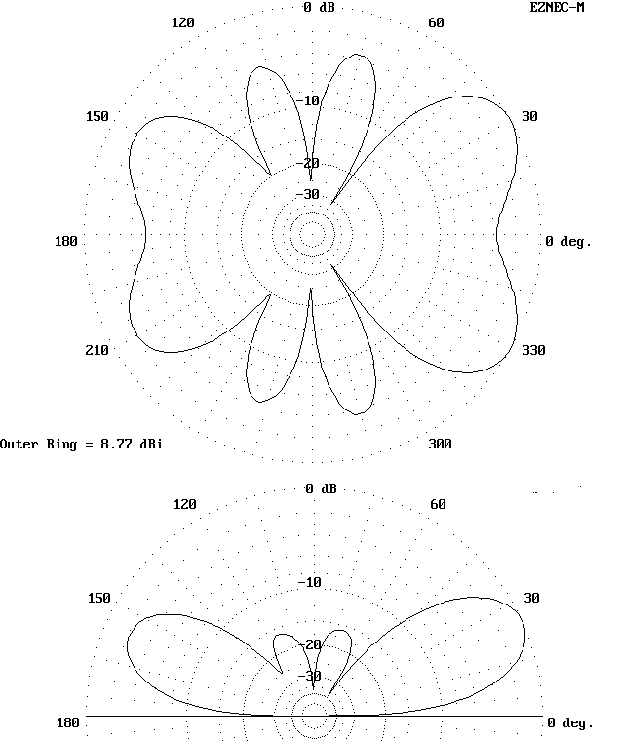#### 17 Meters: 18.1 MHz

100-ohm Connection: Zi = 205 + j15 ohms -- Elevation angle = 20 degrees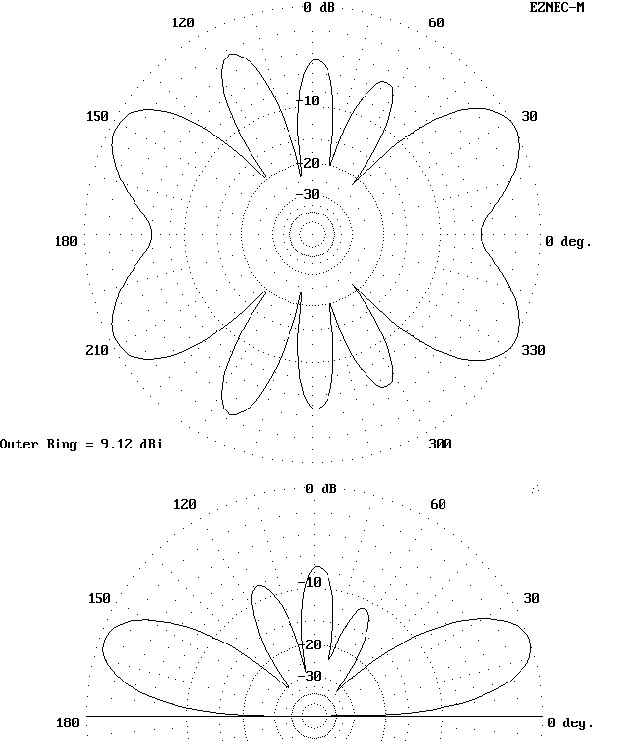Note that the 100-ohm and 300-ohm patterns are again similar, with difference confined to the minor lobes.

300-ohm Connection: Zi = 170 + j65 ohms -- Elevation angle = 19 degrees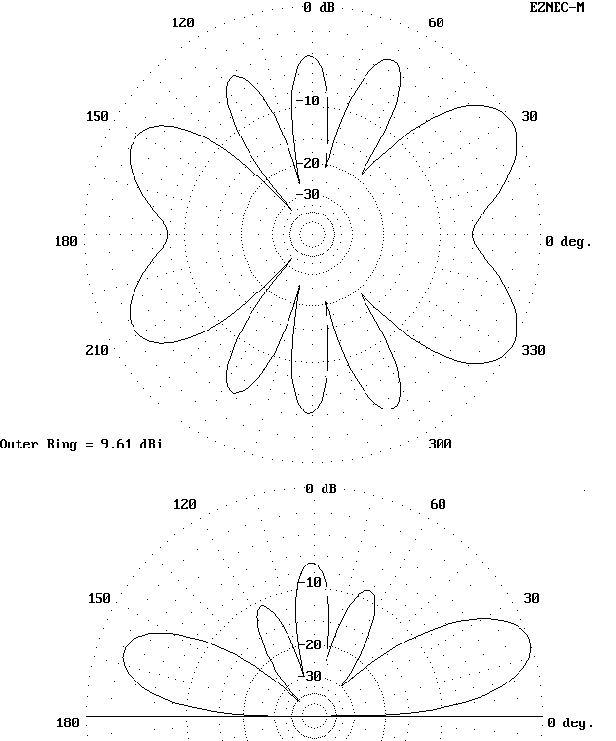#### 15 Meters: 21.15 MHz

100-ohm Connection: Zi = 135 + j240 ohms -- Elevation angle = 17 degrees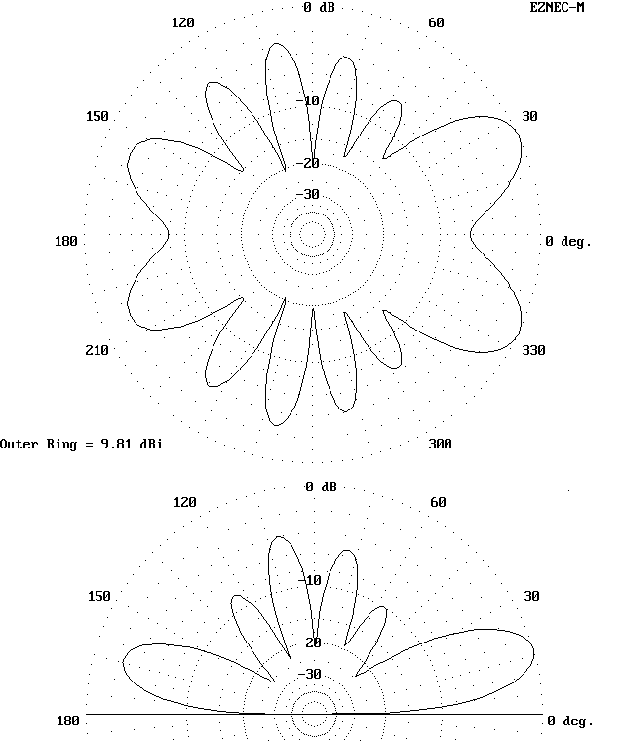On 15 meters, the 300-ohm version of the antenna shows considerably less gain to the short side of the antenna element.

300-ohm Connection: Zi = 920 - j600 ohms -- Elevation angle = 17 degrees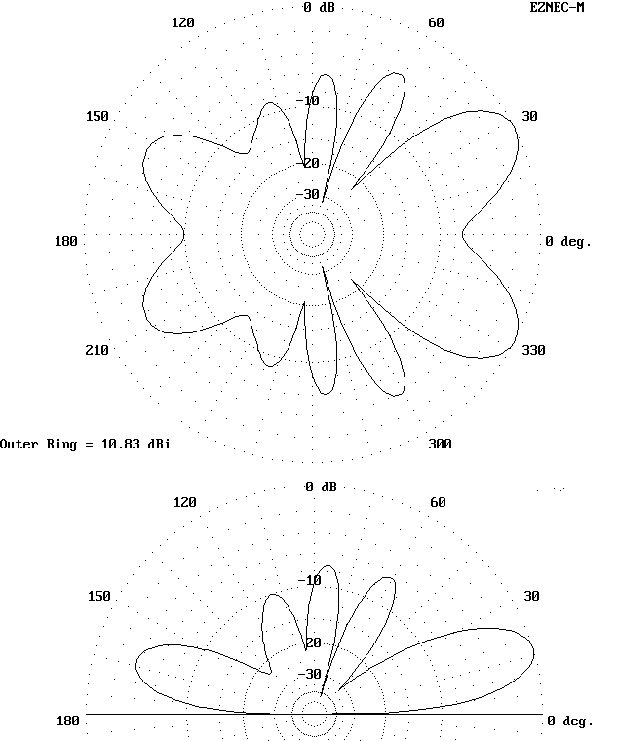#### 12 Meters: 24.95 MHz

100-ohm Connection: Zi = 545 - j420 ohms -- Elevation angle = 15 degrees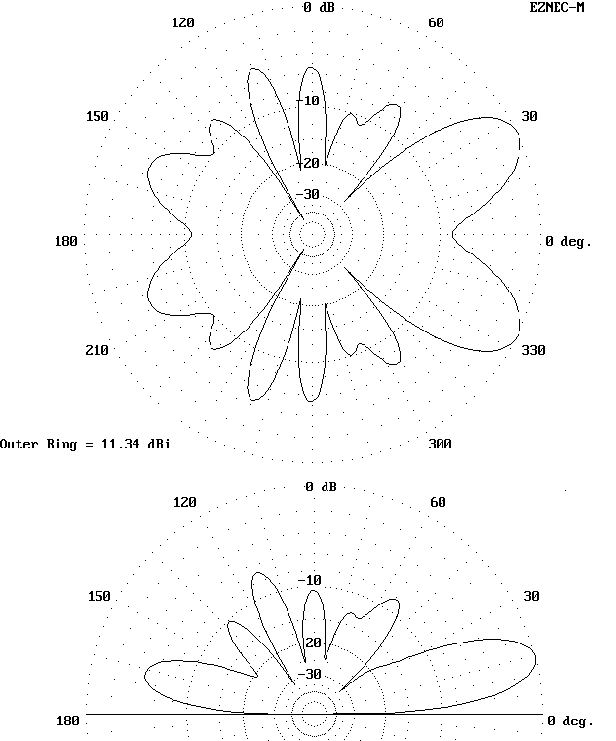Note that, although the number of lobes are the same, the 300-ohm version puts more energy into high lobes and less into lobes off the ends of the antenna.

300-ohm Connection: Zi = 2930 + j350 ohms -- Elevation angle = 15 degrees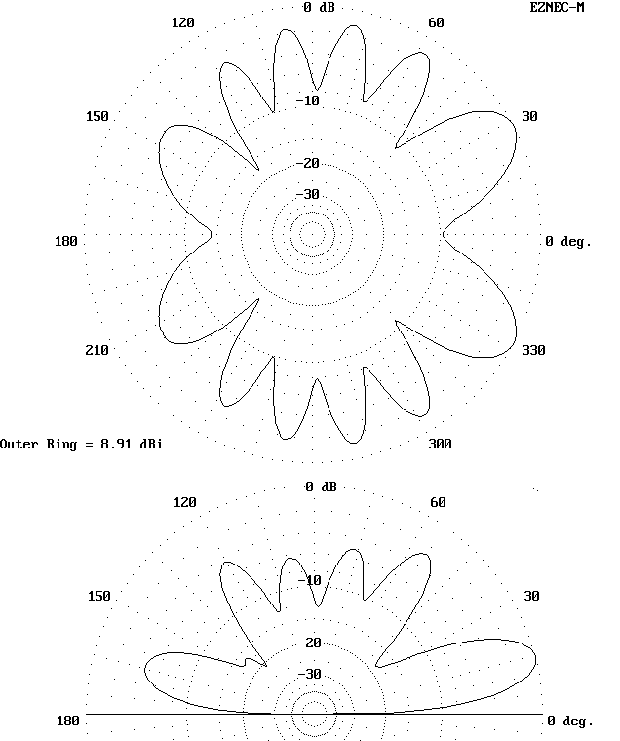#### 10 Meters: 28.5 MHz

100-ohm Connection: Zi = 1755 - j905 ohms -- Elevation angle = 14 degrees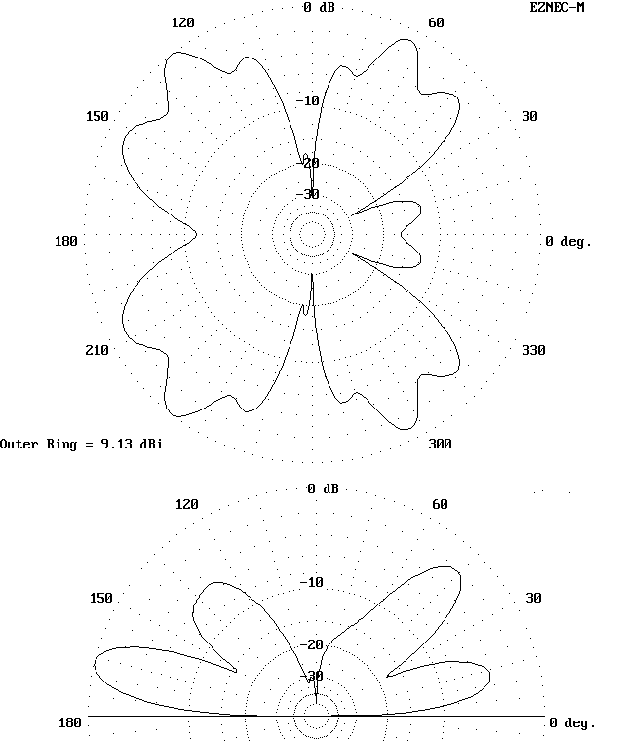Note the deep nulls broadside to the 100-ohm version of the antenna, compared to the smoother lobe structure of the 300-ohm version.

300-ohm Connection: Zi = 875 - j925 ohms -- Elevation angle = 15 degrees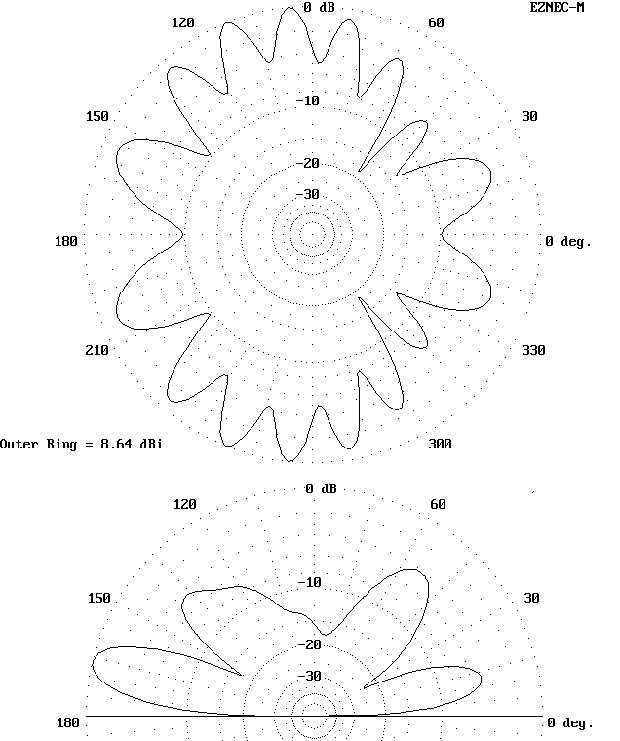Updated 1-20-98. © L. B. Cebik, W4RNL. Data may be used for personal purposes, but may not be reproduced for publication in print or any other medium without permission of the author.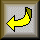Go to series index page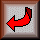Return to Amateur Radio Page